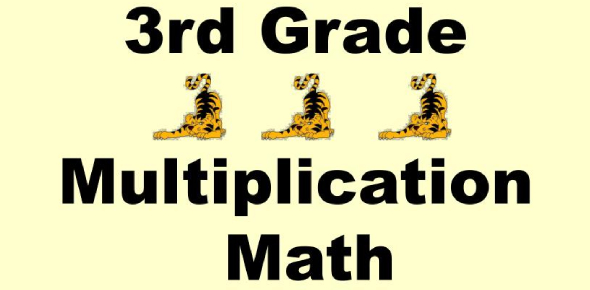# Multiplication Quiz For Grade 3

12 Questions | Attempts: 14185
ShareSettingsMost students in third-grade face problems in solving division and multiplication questions. Given below is a multiplication quiz for grade 3 students that consist of some mathematics problems involving multiplication. Try out this quiz and see how many you can correct answers you can give. This quiz is based on the MCQ pattern, and you need to pick only one most appropriate answer to every question. So, good luck and get started.

• 1.
What is 4 x 2=?
• A.

6

• B.

8

• C.

2

• D.

5

• 2.
What is 5 x 3=?
• A.

12

• B.

8

• C.

10

• D.

15

• 3.
What is 6 x 3=?
• A.

18

• B.

9

• C.

3

• D.

14

• 4.
What is 4 x 1=?
• A.

5

• B.

3

• C.

4

• D.

0

• 5.
What is 3 x 3=?
• A.

9

• B.

0

• C.

6

• D.

4

• 6.
What is 4 x 3=?
• A.

6

• B.

7

• C.

12

• D.

1

• 7.
What is 6 x 2=?
• A.

12

• B.

4

• C.

1

• D.

8

• 8.
What is 4 x 4=?
• A.

8

• B.

0

• C.

16

• D.

12

• 9.
What is 7 x 2=?
• A.

14

• B.

9

• C.

5

• D.

12

• 10.
What is 5 x 2=?
• A.

3

• B.

7

• C.

11

• D.

10

• 11.
What is 1 x 9=?
• A.

8

• B.

10

• C.

9

• D.

12

• 12.
What is 5 x 6=?
• A.

11

• B.

1

• C.

30

• D.

20

## Related TopicsBack to top
×

Wait!
Here's an interesting quiz for you.